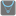• # Apparent bug

## Question related to missionMin and Max

Hi.

I'm having a problem with this puzzle. My code passes the asserts but then an error appears from outside my code. The error is -

TypeError: 'generator' object has no attribute 'getitem', min, 5, , 9 "min(abs(i) for i in range(-10, 10))"

This is the third time I've had one of these puzzles fail. The other two were Python 2/3 issues as the asserts were plain strings but the parameters being passed were unicode, which would have been fine in Python 3 but caused errors in Python 2. I'd recommend that the parameters passed to python 2 code be standard strings or, if that doesn't appeal to you, that at a minimum the fact that the parameters are unicode be added to the parameter specification of the description of the puzzle.

Ian Simcock.

HTTP_USER_AGENT:

```Mozilla/5.0 (Windows NT 6.2; WOW64; rv:30.0) Gecko/20100101 Firefox/30.0
```

My Code:

```def min(*args, **kwargs):
key = kwargs.get("key", None)
sort_source = args if len(args) > 1 else args
min_value = sort_source
if key:
min_key = key(sort_source)
for entry in sort_source[1:]:
new_key = key(entry)
if new_key < min_key:
min_value = entry
min_key = new_key
else:
for entry in sort_source[1:]:
if entry < min_value:
min_value = entry

return min_value

def max(*args, **kwargs):
key = kwargs.get("key", None)
sort_source = args if len(args) > 1 else args
max_value = sort_source
if key:
max_key = key(sort_source)
for entry in sort_source[1:]:
new_key = key(entry)
if new_key > max_key:
max_value = entry
max_key = new_key
else:
for entry in sort_source[1:]:
if entry > max_value:
max_value = entry

return max_value

if __name__ == '__main__':
#These "asserts" using only for self-checking and not necessary for auto-testing
assert max(3, 2) == 3, "Simple case max"
assert min(3, 2) == 2, "Simple case min"
assert max([1, 2, 0, 3, 4]) == 4, "From a list"
assert min("hello") == "e", "From string"
assert max(2.2, 5.6, 5.9, key=int) == 5.6, "Two maximal items"
assert min([[1, 2], [3, 4], [9, 0]], key=lambda x: x) == [9, 0], "lambda key"
```